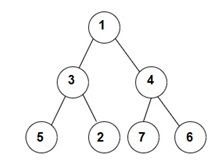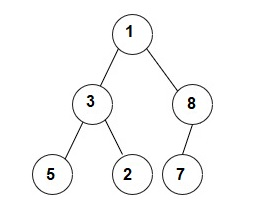New update is available. Click here to update.

# Binary Tree Zigzag Traversal

Last Updated: 31 Dec, 2020
Difficulty: Easy

## PROBLEM STATEMENT

#### You have been given a Binary Tree of 'N' nodes, where the nodes have integer values. Your task is to print the zigzag traversal of the given tree.

##### Note:
``````In zigzag order, level 1 is printed from left to right fashion, level 2 is printed from right to left. and level 3 is printed from left to right again, and so on…..
``````
##### For example:
``````For the given binary tree
````````````The zigzag  traversal is [1, 4, 3, 5, 2, 7, 6]
``````
##### Input Format:
``````The first line contains an integer 'T' which denotes the number of test cases or queries to be run. Then the 'T' test cases follow.

The first line of each test case contains elements of the tree in the level order form. The line consists of values of nodes separated by a single space. In case a node is null, we take -1 in its place.

For example, the input for the tree depicted in the below image
````````````1
3 8
5 2 7 -1
-1 -1 -1 -1 -1 -1
``````

#### Explanation :

``````Level 1 :
The root node of the tree is 1

Level 2 :
Left child of 1 = 3
Right child of 1 = 8

Level 3 :
Left child of 3 = 5
Right child of 3 = 2
Left child of 8 =7
Right child of 8 =  null (-1)

Level 4 :
Left child of 5 = null (-1)
Right child of 5 = null (-1)
Left child of 2 = null (-1)
Right child of 2 = null (-1)
Left child of 7 = null (-1)
Right child of 7 = null (-1)

The first not-null node(of the previous level) is treated as the parent of the first two nodes of the current level. The second not-null node (of the previous level) is treated as the parent node for the next two nodes of the current level and so on.
The input ends when all nodes at the last level are null(-1).
``````
##### Note :
``````The above format was just to provide clarity on how the input is formed for a given tree.
The sequence will be put together in a single line separated by a single space. Hence, for the above-depicted tree, the input will be given as:
1 3 8 5 2 7 -1 -1 -1 -1 -1 -1 -1
``````
##### Output Format:
``````For each test case, print a single line containing all the nodes value in zigzag order traversal separated by a single space in a separate line.
``````
##### Note :
``````You do not need to print anything, it has already been taken care of. Just implement the given function.
``````
##### Constraints:
``````1 <= 'T' <= 100
0 <= 'N' <= 10^3
0 <= 'NODES' <= 10^9

Where NODES represent any node value

Time Limit: 1sec
``````## Approach 1

We can use level order traversal (recursive) to explore all levels of the tree. Also, at each level nodes should be printed in alternating order.

For example - First level of tree should be printed in left to right manner, Second level of tree should be printed in right to left manner, Third again in left to right order and so on.

So, we will use a Direction variable whose value will toggle at each level and we will print levels in alternating order by looking at the direction of current level.

We will first calculate the maximum level of the binary tree, then we will run a loop from 1 to maximum level and store nodes of each level in our answer based on direction.

If direction is 0 then traverse the whole binary tree from left to right and store nodes of current level in left to right fashion.

If direction is 1 then traverse the whole binary tree from right to left and store nodes of current level in right to left fashion.